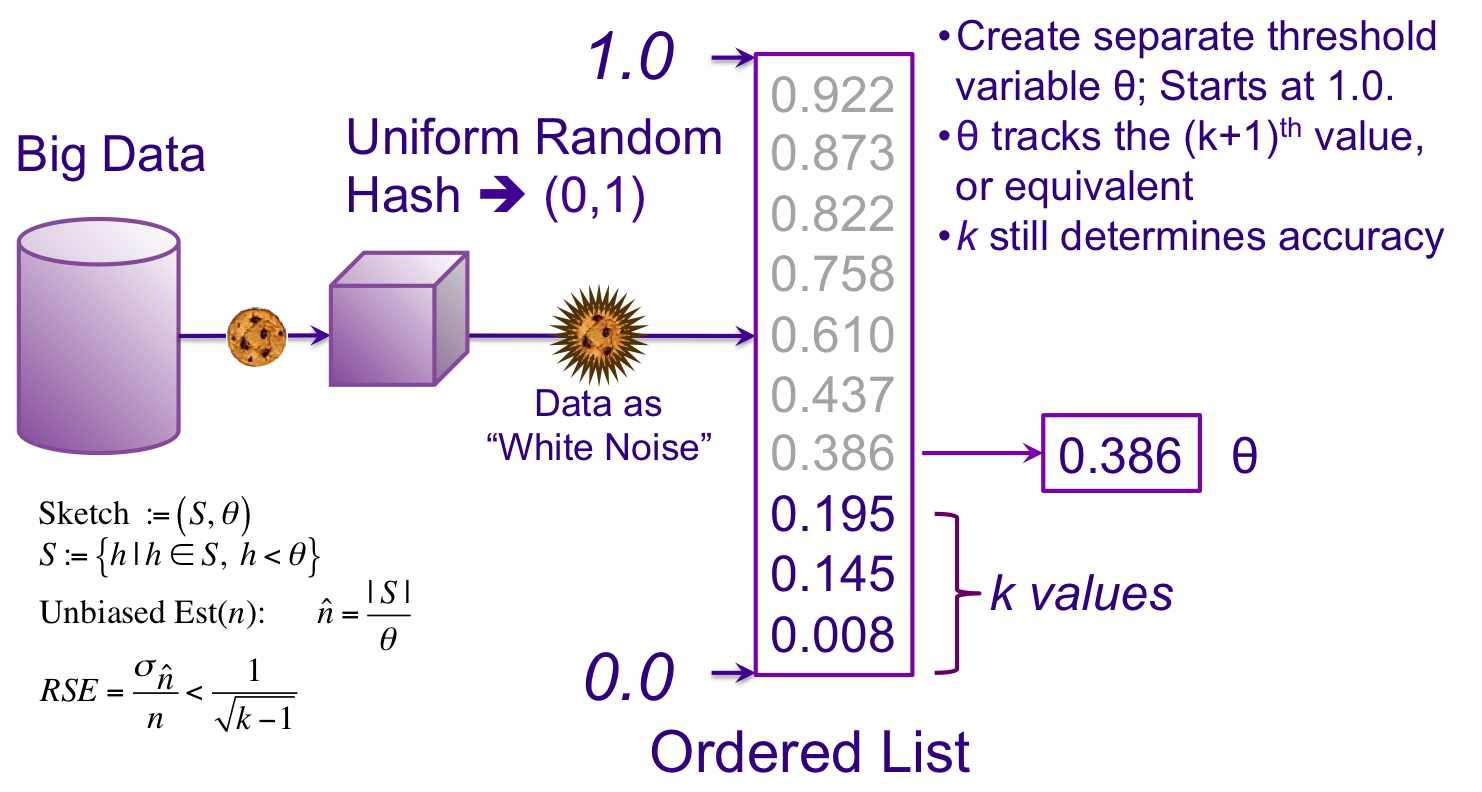## Theta Sketch Framework

Theta Sketches are a generalization of the well known Kth Minimum Value (KMV)1,2 sketches in that KMV sketches are a form of Theta Sketch, but not all Theta Sketches are KMV.

The Theta Sketch Framework (TSF) is a mathematical framework defined in a multi-stream setting that enables set expressions over these streams and encompasses many different sketching algorithms. A rudimentary introduction to the mathematics of the simpler sketch algorithms is developed in the Theta Sketch Equations document.

The TSF consists of the following components:

1. A data type (θ,S), known as a Theta Sketch, where 0 < θ < 1 is a threshold, and S, the number of entries, is the set of all unique hashed stream items 0 < x < 1 that are less than θ.
2. A universal “combining function” ThetaUnion that takes as input a collection of Theta Sketches and returns a single Theta Sketch that is a Union of the input sketches. This combining function is extended to set Intersections and Differences as well.
3. A estimator function that takes as input a Theta Sketch and returns an estimate of the unique hashed stream items presented to all the input sketches.

The TSF enables this sketch library to encompass multiple sketching algorithms including the KMV sketch with a common API and greatly simplifies implementation of set expressions.

Note that in the KMV sketch the value k is overloaded with multiple roles:

1. k determines the RSE (accuracy) of the sketch
2. k determines the upper-bound size of the sketch
3. k is used as a constant in the estimator and RSE equations
4. k determines the V(kth) threshold, used to reject/accept hash values into the cache.

By unloading some of these roles, we will gain degrees of freedom to do some innovative things.

Instead of having to track V(kth), which is a member of the list of hash values, we are going to create a separate threshold variable and call it theta (θ). This effectively decouples #3 and #4 above from k. When the sketch is empty θ = 1.0. After the sketch has filled with k minimum values θ is still 1.0. When the next incoming unique value must be inserted into the sketch the (k+1)th minimum value, is assigned to θ and removed from the cache.3

Ultimately, it will be the size of S, |S|, that will determine the stored size of a sketch, which decouples #2 above from the value k. The Nominal Entries or k is a user specified, configuration parameter, which is used by the software to determine the target accuracy of the sketch and the maximum size of the sketch.

The unbiased estimate simplifies to |S|/θ, which is just the size of S divided by θ. We will discuss the RSE in a later section.Z. Bar-Yossef, T. Jayram, R. Kumar, D. Sivakumar, and L. Trevisan. Counting distinct elements in a data stream. In Randomization and Approximation Techniques in Computer Science, pages 1–10. Springer, 2002.

 See KMV Tutorial and Sketch of the Day: K-Minimum Values for a brief tutorials on KMV Sketches.

 This is a limited “KMV perspective” on how θ gets assigned. The attached paper Theta Sketch Framework presents multiple ways that θ can be assigned using the Theta Choosing Function (TCF). Different sketch algorithms have different TCFs.